Get instant live expert help with Excel or Google Sheets“My Excelchat expert helped me in less than 20 minutes, saving me what would have been 5 hours of work!”

#### Post your problem and you'll get expert help in seconds

Your message must be at least 40 characters
Our professional experts are available now. Your privacy is guaranteed.

# How to Use the Excel Sum Function in Google Sheets and Excel

We can sum entire columns in Excel using the SUM function. The SUM function takes numbers and sums them up. In this tutorial, we will learn how to add a whole column in Excel using the SUM function.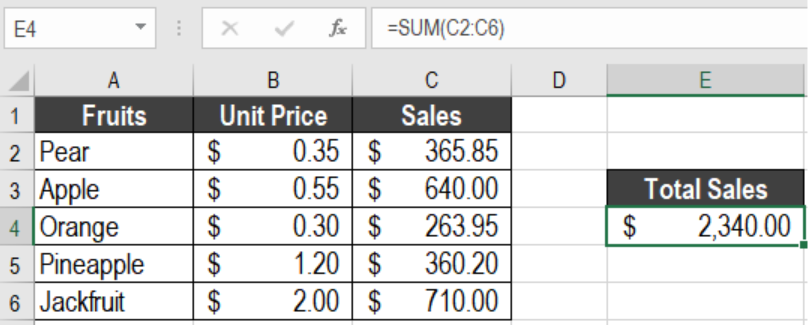Figure 1. Example of How to Sum Entire Column in Excel

## Syntax

`=SUM(num1, [num2],...)`

## Arguments

• number1 – This argument is required. The first number. Excel can sum numbers, cell references, ranges, arrays, and constants. It can also sum the output of other formulas or functions.
• number2 – This argument is optional. It is the second number to sum. Excel can take up to 255 arguments to sum.

## How to Total Columns in Excel

In this example, we will be using a fruit sales database. Columns A, B and C have the names, unit price and sales.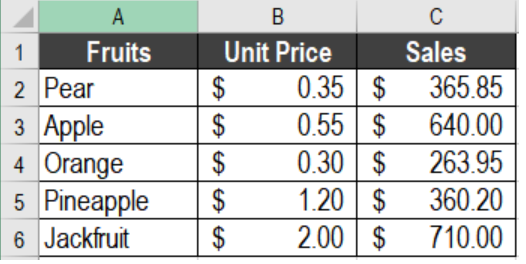Figure 2. The Fruit Sale Database Used in the Tutorial

We will calculate the sumof the sales column. To find the Total sales in cell E4, we need to:

• Click on cell E4.
• Next, we need to go to the Formulas tab on the ribbon. Then, we click Insert formulas.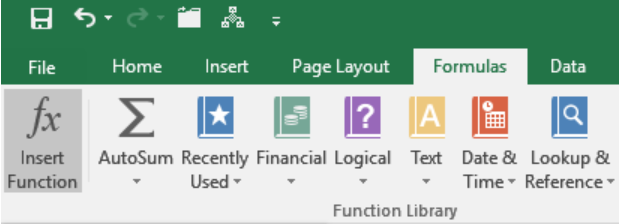Figure 3. The Insert Function Option

• In the Search for a Function box of the Insert Function, we have to type SUM. Click Go.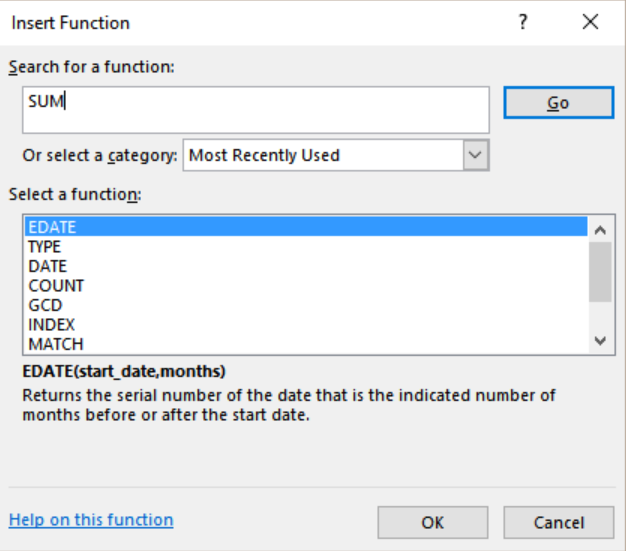Figure 4. Searching the SUM Function

• You will see the SUM function in the Select a function list. Click on it. Press Ok.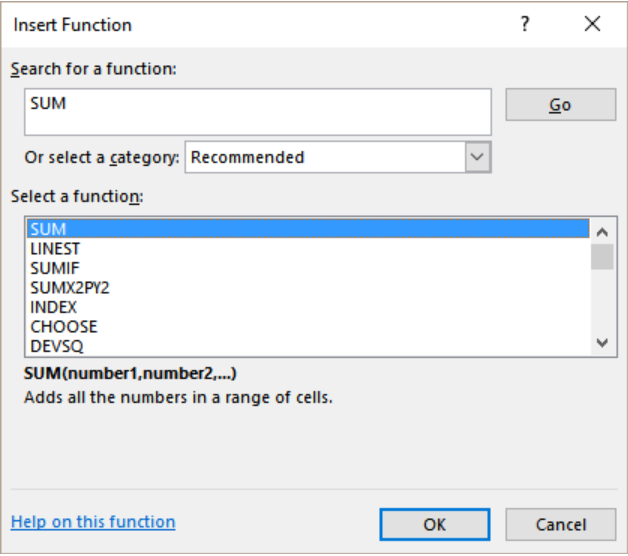Figure 5. Selecting the SUM Function

• This will open Function Argument box for the SUM function. Click on the right spreadsheet icon on the right of the arguments for B1.
• This will open up a box to set the range for B1.
• Now we need to click on C2 and drag the selection from C2 to C6.
• Click on the spreadsheet icon again. This will take us back to the Function Argument box.
• Finally, we need to click Ok.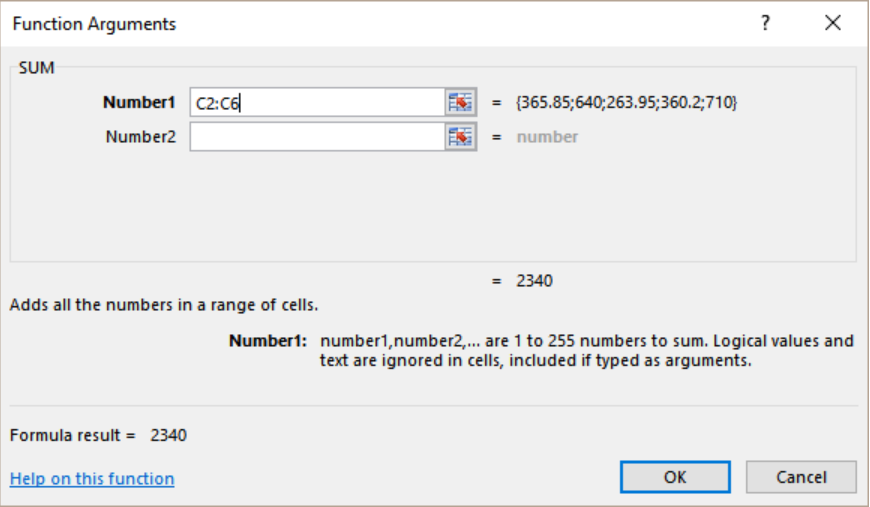Figure 6. Example of Setting the Parameters of the SUM Function

This will show the sum of the column sales in cell E4.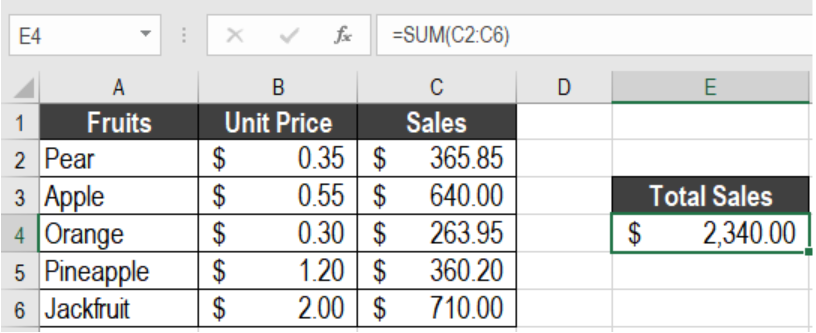Figure 7. Totaling in Excel with the SUM Function

## Using the Excel Sum Function in Google Sheets

We can use the SUM function in a similar way in google sheets. To do the previous example in Google Sheets,

• We need to go to cell E4.
• Click on Insert > Function > Math > Sum.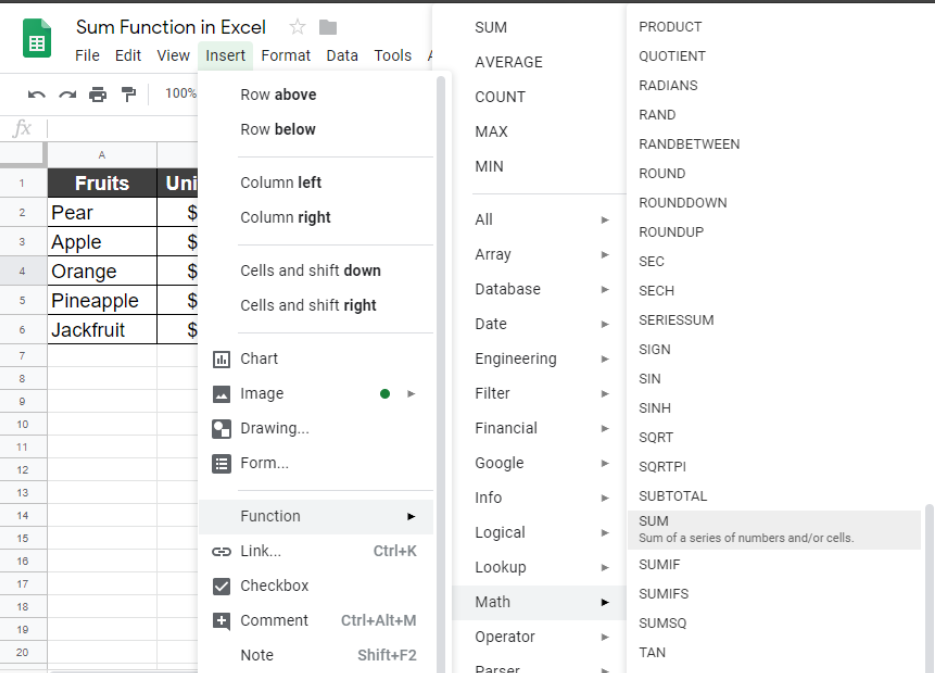Figure 8. The SUM Function in Google Sheets

• This will insert the SUM function in cell E4. Now, we have to set the range.
• To do that, we need to click on C2 and drag the selection from C2 to C6.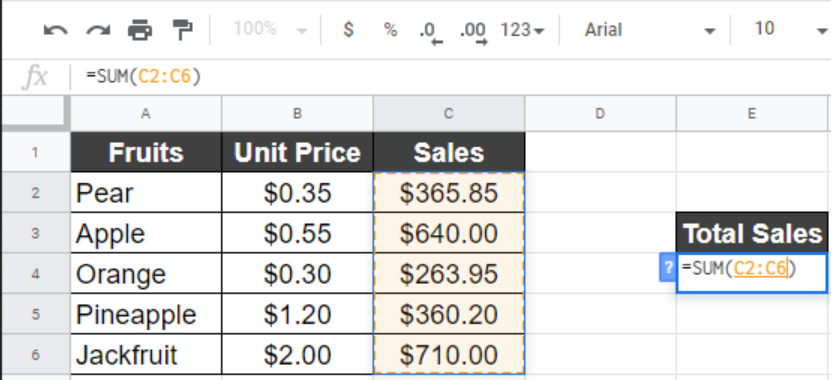Figure 9. Assigning the Formula in Google Sheets

• Finally, we need to click Enter.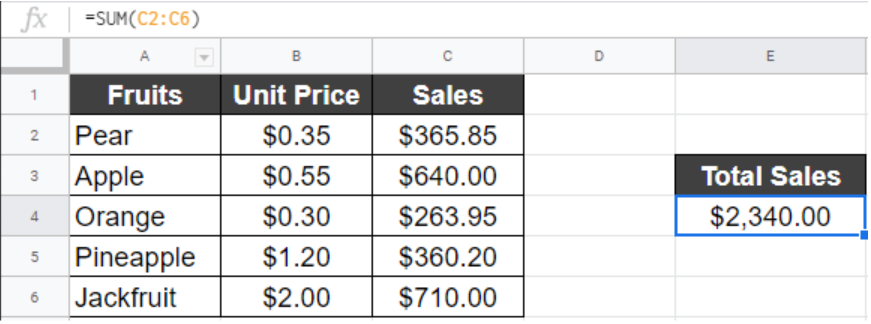Figure 10. Example of How to Add a Whole Column in Google Sheets

This will show the sum of column C in cell E4, which is \$2340.00.

Most of the time, the problem you will need to solve will be more complex than a simple application of a formula or function. If you want to save hours of research and frustration, try our live Excelchat service! Our Excel Experts are available 24/7 to answer any Excel question you may have. We guarantee a connection within 30 seconds and a customized solution within 20 minutes.

### Did this post not answer your question? Get a solution from connecting with the expert.Another blog reader asked this question today on Excelchat:
Related blogs## Subscribe to Excelchat.coAnother blog reader asked this question today on Excelchat: